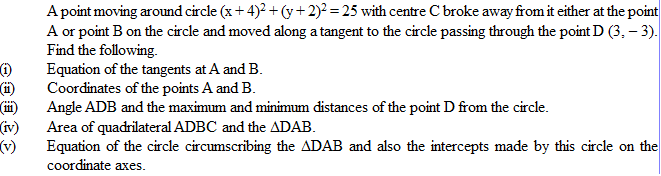# circle 1•(i) 4y-3x+21=0 and 3y-4x-3=0
(ii) (-1, -6) and (0, 1)
(iii) angle ADB = 90Â°; max distance = 5(âˆš2+1) units, min distance = 5(âˆš2-1) units
(iv) Area of ADBC = 25 square units; area of DAB = 12.5 square units
(v) x2+y2+x+5y-6=0; intercept on x-axis = 7 units, intercept on y-axis = 5 units.

•Manish Shankar ·